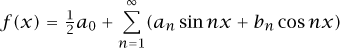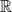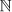Definition: Cantor, Georg from The Columbia Encyclopedia

(gā'ôrkh kän'tôr), 1845–1918, German mathematician, b. St. Petersburg. He studied under Karl Weierstrass and taught (1869–1913) at the Univ. of Halle. He is known for his work on transfinite numbers and on the development of set theory, which is the basis of modern analysis, as well as for his definition of irrational numbers. His approach to the concept of the infinite revolutionized mathematics by challenging the processes of deductive reasoning and led to a critical investigation of the foundations of mathematics.

Summary Article: VI.54 Georg Cantor
From The Princeton Companion to Mathematics

Although born in Russia, Cantor was raised and educated in Prussia and spent his entire career as professor of mathematics at the University of Halle. He studied at the Universities of Berlin and Göttingen with kronecker [VI.48], kummer [VI.40], and weierstrass [VI.44], and received his Ph.D. from the University of Berlin in 1867. His dissertation, “De aequationibus secundi gradus indeterminatis” (“On indeterminate equations of the second grade”), dealt with work in number theory on Diophantine equations, work that had been pioneered by lagrange [VI.22], gauss [VI.26], and legendre [VI.24]. The following year he accepted a position in the mathematics department at the University of Halle, where he spent the rest of his academic career. There, his Habilitationsschrift was again devoted to number theory, and dealt with transformations of ternary quadratic forms.

It was at Halle that Cantor’s colleague, Eduard Heine, was working on difficult problems involving trigonometric series, and he interested Cantor in the problem of determining the conditions under which a trigonometric series of the formuniquely represented a given function. In other words, could it be that two different trigonometric series could represent the same function? Heine had shown, in 1870, that if f(x) is continuous in general (i.e., for all but a finite number of points of discontinuity, at which points Heine added that the function need not necessarily be finite), the representation is unique if we insist that the series is uniformly convergent to f in general. Cantor was able to establish much more general results, and in five papers written between 1870 and 1872 he was able to show that such representations were unique even if an infinite number of exceptional points were allowed, so long as these exceptional points (i.e., points at which the function failed to be continuous) were distributed over the domain of the function’s definition in a particular way, constituting what Cantor called “point sets of the first species.” His studies of these and related point sets eventually led Cantor to his much more abstract and powerful theory of sets and transfinite numbers.

Point sets of the first species were sets P for which, given its sequence of derived sets (the derived set P′ of a set P is the set of all the limit points of P), there was some finite n such that the nth derived set Pn of P was finite, and thus the (n + 1)st derived set was empty, i.e., Pn+1 = ∅. It was Cantor’s subsequent study of infinite linear point sets that would eventually lead to his creation of transfinite set theory in the 1880s. (For more details about this, see set theory [IV.22 §2].)

Before he did so, Cantor first began to explore the implications of his work on trigonometric series and the structure of the real numbers in several papers, one of which was to revolutionize mathematics in a fundamental way. The first of these papers was published in 1874, and bore the innocuous title “Über eine Eigenschaft des Inbegriffes aller reellen algebraischen Zahlen” (“On a property of the collection of all real algebraic numbers”). In this paper, Cantor proved that the set of all algebraic real numbers was countably infinite [III.11]. What was revolutionary about the paper, however, was that he also proved that the set of all real numbers was not countable, and must be of a higher order of infinity than the countably infinite set of natural numbers. He returned to this result in 1891 with a new approach, the groundbreaking method of diagonalization, to prove in a very direct way that the set of real numbers is uncountably infinite. Cantor’s second important paper of the decade appeared in 1878, “Ein Beitrag zur Mannigfaltigkeitslehre” (“A contribution to the theory of aggregates”), in which he proved (with a partly faulty argument) the invariance of dimension, a theorem first correctly proved by brouwer [VI.75] in 1911.

Between 1879 and 1884 Cantor published six papers designed to outline the basic elements of his new thinking about sets. He first considered what happened if a set were not of the first species by introducing symbols for the infinite indices needed to identify such sets. For example, a set P was said to be of the second species if there was no finite n such that the nth derived set Pn of P was finite. He then considered the case in which the intersection of all the derived sets of P (namely P′, P″, . . ., Pn,. . .) was again an infinite set, which he designated P. This set, since it was infinite, had a derived set as well, P∞+1, and this led in fact to an entire sequence of derived sets of the second species: P, P∞+1,. . ., P∞+n, . . ., P2∞,. . ..

In his first papers on infinite linear sets, these indices for derived sets remained “infinitary symbols”: that is, devices for distinguishing between different sets. But in his Grundlagen einer allgemeinen Mannigfaltigkeitslehre (“Foundations of a general set theory”), published in 1883, these symbols became the first transfinite numbers: the transfinite ordinal numbers. These numbers began with ω, the transfinite ordinal number representing the sequence of natural numbers 1, 2, 3,. . ., which could also be thought of as the first infinite ordinal number after all of the finite whole numbers. In the Grundlagen, Cantor not only devised the basic features of a transfinite arithmetic for these numbers, but he provided a detailed philosophical defense of the new numbers. Acknowledging the revolutionary nature of what he was introducing, he argued that the new concepts were necessary in order to achieve precise mathematical results that he could obtain by no other means.

Cantor’s best-known mathematical creation, however, the transfinite cardinal numbers, which he denoted using the Hebrew letter aleph, were introduced only later, in the 1890s. They were first given full exposition in a pair of papers (1895, 1897) that constituted his “Beiträge zur Begrundung der transfiniten Mengenlehre” (“Contributions to the founding of transfinite set theory”). In two articles published in Mathematische Annalen, he not only set out his theory of transfinite ordinal and cardinal numbers, as well as their arithmetics, but also explained his theory of order types, namely the different properties exhibited by the sets of natural, rational, and real numbers considered in their natural orders. There he also stated (but could not prove) his famous continuum hypothesis [IV.22 §5], namely that the power (or cardinal number) of the continuum of all real numbersis the next largest infinite set (or cardinal number) after the countably infinite set of natural numbers, the cardinality of which was taken to be א0. Cantor expressed the continuum hypothesis algebraically as the statement that 2א0 = א1.

By the end of his career Cantor had received honorary degrees from foreign universities and the Copley Medal of the Royal Society for his great contributions to mathematics, but there were problems with set theory that were beyond his capacities to remedy. The most disturbing for many mathematicians were the “antinomies” of set theory: the paradoxes put forward by the likes of Burali-Forti and russell [VI.71]. In 1897 the former published the paradox arising from the collection of all ordinal numbers, the ordinal number of which should be an ordinal number greater than any in the collection of all ordinal numbers. In 1901 the latter discovered the paradox of the class of all classes that are not members of themselves: is it a member of itself or not? (See the crisis in the foundations of mathematics [II.7 §2.1].) Cantor himself was aware of the contradictions that arose from considering the collections of all transfinite ordinal or cardinal numbers, and what their ordinal or cardinal numbers might be. The solution Cantor adopted was to regard such collections as too large, and not really sets at all, but “inconsistent aggregates” as he called them. Others, like Zermelo, began to axiomatize set theory in an effort to exclude the possibility of contradictions. The two most powerful results of the twentieth century to complement Cantor’s work are those of gödel [VI.92] (who established the consistency of the continuum hypothesis with zermelo–fraenkel set theory [IV.22 §3]) and Paul Cohen (who determined the independence of the continuum hypothesis from Zermelo–Fraenkel set theory), the latter finally establishing the impossibility of proving the continuum hypothesis.

Cantor’s legacy for the history of mathematics has truly been revolutionary. Above all, his transfinite set theory for the first time gave mathematicians the means of dealing with concepts of the infinite in a careful and precise way.

• 1990. Georg Cantor. His Mathematics and Philosophy of the Infinite. Princeton, , NJ: Princeton University Press. (First published in 1978 by Harvard University Press.).
• 2005. Georg Cantor and the battle for transfinite set theory. In Kenneth O. May Lectures of the Canadian Society for History and Philosophy of Mathematics, edited by and , pp. 221-41. New York: Springer.
• 2005. Georg Cantor. Paper on the “Foundations of a general set theory” (1883). In Landmark Writings in Western Mathematics 1640-1940, edited by , pp. 600-12. London: Routledge.
• 2005. Kardinalität und Kardinäle. Wissenschaftshistorische Aufarbeitung der Korrespondenz zwischen Georg Cantor und katholischen Theologen seiner Zeit. Stuttgart: Franz Steiner.

•Copyright © 2010 by Princeton University Press

### Related Articles

##### Full text Article Cantor, Georg (1845 - 1918)
The Macmillan Encyclopedia

Cantor's family moved in 1856 to Germany, where he spent the rest of his life. He was the first mathematician to set the...

##### Full text Article Cantor, Georg
Biographical Dictionary of 20th Century Philosophers

German, b: 3 April 1845, St Petersburg, Russia, d: 6 January 1918, Halle, Germany. Cat: Mathematician, Ints: Philosophy of mathematics. ...

##### Full text Article Cantor, Georg 1845-1918
Reader's Guide to the History of Science

Russian-born German mathematician Bell E. T. , Men of Mathematics, New York : Simon and Schuster , 1937 ; Harmondsworth : ...

See more from Credo